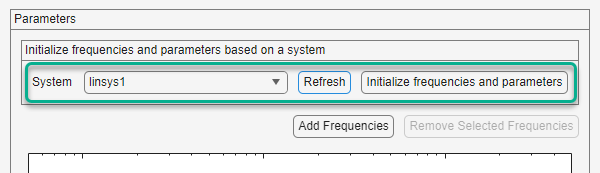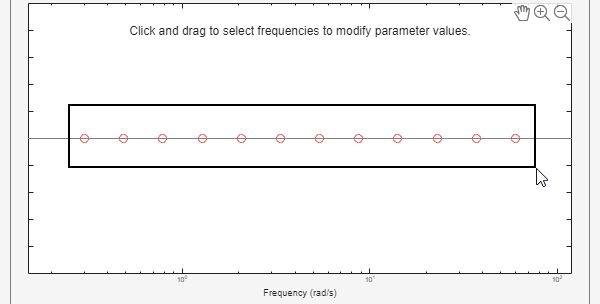## Validate Linearization In Frequency Domain

### Validate Linearization in Frequency Domain using Model Linearizer

This example shows how to validate linearization results using an estimated linear model.

In this example, you linearize a Simulink® model using the I/Os specified in the model. You then estimate the frequency response of the model using the same operating point (model initial condition). Finally, you compare the estimated response to the exact linearization result.

1. Open the model.

```sys = 'scdDCMotor'; open_system(sys)```
2. Open the Model Linearizer for the model.

In the Simulink model window, in the Apps gallery, click Model Linearizer.

3. Linearize the model at the default operating point and analysis I/Os, and generate a bode plot of the result.

ClickBode. The Bode plot of the linearized plant appears, and the linearized plant `linsys1` appears in the Linear Analysis Workspace.

Estimate Frequency Response of Model

1. Create a sinestream input signal for computing an approximation of the model by frequency response estimation. In the Estimation tab, in the Input Signal drop-down list, select `Sinestream`.

2. Initialize the input signal frequencies and parameters based on the linearized model.

Click .The frequency display in the dialog box is populated with frequency points. The software chooses the frequencies and input signal parameters automatically based on the dynamics of `linsys1`.

3. Set the amplitude of the input signal at all frequency points to 1. In the frequency display, select all the frequency points.Enter 1 in the Amplitude field, and click . The new input signal `in_sine1` appears in the Linear Analysis Workspace.

4. Estimate the frequency response and plot its frequency response on the existing Bode plot of the linearized system response. ClickBode Plot 1.

Examine estimation results.

Bode Plot 1 now shows the Bode responses for the estimated model and the linearized model.The frequency response for the estimated model matches that of the linearized model.

### Choosing Frequency-Domain Validation Input Signal

For frequency-domain validation of linearization, create a sinestream signal. By analyzing one sinusoidal frequency at a time, the software can ignore some of the impact of nonlinear effects.

SinestreamAll linearization inputs and outputs are on continuous signals.`frest.Sinestream`
Sinestream with fixed sample timeOne or more of the linearization inputs and outputs is on a discrete signal`frest.createFixedTsSinestream`

You can easily create a sinestream signal based on your linearized model. The software uses the linearized model characteristics to accurately predict the number of sinusoid cycles at each frequency to reach steady state.

When diagnosing the frequency response estimation, you can use the sinestream signal to determine whether the time series at each frequency reaches steady state.

## Related Topics